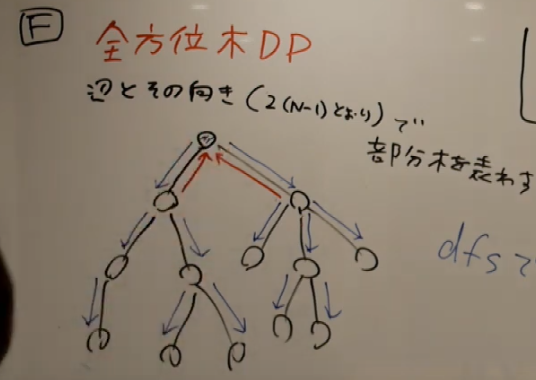# AtCoder Beginner Contest 160

Updated:

AtCoder Beginner Contest 160

# Solutions

## A - Coffee

Check if $S = S \land S = S$ or not.

## B - Golden Coins

Use division and modulo operators. Greedy algorithm works.

## C - Traveling Salesman around Lake

Fortunately, the input is already sorted. The answer is $K$ minus the maximum of the distance between the house $i$ and $i + 1$. We can calculate this maximum as follows.

  int maxi{A + K - A[N - 1]};
for (auto i{0}; i < N - 1; ++i)
{
ch_max(maxi, A[i + 1] - A[i]);
}


## D - Line++

This graph is almost a line. Let $(i, j) \in N ^ 2$ with $i < j$. Then, the distance without the edge $(X, Y)$ is $\lvert j - i \rvert$.

We think of the edge $(X, Y)$. If we use this edge, the tour from $i$ to $j$ is either of the followings: $i \mapsto X \mapsto Y \mapsto j$ or $i \mapsto Y \mapsto X \mapsto i$. The distances of the former is $\lvert i - X \rvert + 1 + \lvert Y - j \rvert$. The distances of the latter is $\lvert i - Y \rvert + 1 + \lvert X - j \rvert$. Thus, the resulting distance between $i$ and $j$ is as follows. $\mathrm{dist}(i, j) = \min \{ \lvert j - i \rvert, \lvert i - X \rvert + 1 + \lvert Y - j \rvert, \lvert i - Y \rvert + 1 + \lvert X - j \rvert \}.$

We make the histogram of above and flush it.

## E - Red and Green Apples

### Observation

First, we ignore the colors. Of course, the greedy algorithm works. We pick up the apples in descending order of the values.

Then, what is the difference between this version and the original problem? The answer is that we cannot eat either more than $X$ red apples or more than $Y$ green apples. Conversely, if we don’t break these limits, it is valid to eat them.

Hence, this problem becomes as follows.

Problem E.1: We have $A$ red apples, $B$ green apples and $C$ colorless apples. We cannot eat either more than $X$ red apples or more than $Y$ green apples. We are going to eat $X + Y$ apples. Find the maximum possible sum of the value of the eaten apples.

### Solution

First, sort $A$ and $B$ in the descending order.

We erase $i$-th elements for $i \geq X$ in $A$ and erase $i$-th elements for $i \geq Y$ in $B$. They are no longer eaten.

Then, merge red, green and colorless apples and sort in the descending order. The answer is the sum of the value of the front $X + Y$ apples.

## F - Distributing Integers

### The version where the root is fixed

First, we fix the root $v$. We solve this problem by tree DP. Suppose that we already know information of the children of $v$. Let $dp[v]$ and $s[v]$ be the answer and the size of the subtree whose root is $v$, respectively. We write $1$ at $v$ first and then we distribute $\{ 2, 3, \dots, s[v] \}$ to the children. We solve the divided problem for children after that. Therefore, we can calculate as follows. \begin{align} s[i] &= 1 + \sum _ {j \in children(i)} s[j], \tag{F.1} \\ dp[i] &= \frac{\left( s[i] - 1 \right)!}{\prod _ {j \in children(i)} s[j]!} \prod _ {j \in children(i)} dp[j] \\ &= (s[i] - 1)! \times \prod _ {j \in children(i)} \frac{dp[j]}{s[j]!}. \tag{F.2} \end{align}

We can solve this problem by Re-rooting Tree DP.

### Re-rooting Tree DP

We solve this problem not only for each vertex but also for each directed edge. We regard the directed edges whose source is $v$ and destination is $w$ is the subtree of $v$ whose root is $w$.We fix one vertex as the root of the following argument. We execute two types of DFS.

#### DFS for normal edges

First, we execute DFS for the edges whose source is the root. This is typical DP calculating (F.1) and (F.2). This works in $O(N)$-time.

#### DFS for vertexes and reversed edges

Second, we execute DFS for the root. This is also the same to calculate (F.1) and (F.2).

Finally, we calculate (F.1) and (F.2) for the reversed edge and pass DFS for the children.

Let $e = (v, w)$ be the normal edge and $e’$ be the reversed edge. Let $D$ and $S$ be the resulting value of $dp[v]$ and $s[w]$ for this vertex $v$, respectively. We can calculate $s[e’]$ and $dp[e’]$ as follows. \begin{align} s[e’] &= S - s[e], \\ dp[e’] &= D \times \frac{\left( s[e’] - 1 \right)!}{\left( S - 1 \right)!} \times \frac{s[e]!}{dp[e]}. \end{align}

We estimate the execution time. The total time spent to calculate DFS for each edges is $O(2 N) = O(N)$. For each reversed edge, the calculation time is $O(1)$. This is an important point. Hence, the total time complexity is $O(N)$.

Tags:

Categories: